# Accident Languages

Some commonly used languages have been quite well designed or at least would have been considered so at the time when they appeared. Even if they have their weaknesses, they should be good for some purposes.

Now beauty of programming languages is highly subjective. So I do not claim any universal truth to this. But I consider the popularity of certain languages as an accident, at least for the time when they became highly popular. This does not preclude that good stuff has been done with them or that they have matured to some extent or even that their potential replacements of the time of their appearance have lost relevance. I do not mention the classics COBOL and Fortran, because they were early pioneers of high level languages. And looking at these two languages, one of them does not deserve to be mentioned together with the other one.

Anyway, here we go:

## Pascal

Pascal was in certain areas popular before C took off. To a great extent this was true because it was used as a teaching language and because Turbo Pascal ran well on a PC with 4.77 MHz and 256k Memory and Floppy Disks only in the early 1980s. This was the hardware that many people could afford with some pain (prices around 5000 DEM in Germany). And it transported some interesting concepts to a wider public. People who knew Algol 68 used to consider it a step backwards. Anyway, in the later 1980s C was available to a broader public, because affordable hardware that could run C-compilers reasonably fast and conveniently came into existence. C does have its flaws, but it is a well designed language and I personable consider the step from Pascal to C one of the most positive ever encountered.

## Python

Python is a good language with a lot of power. But I see it in a similar space as Ruby, which to me appears as one of the most beautifully designed languages. So why use Python if Ruby could serve the same purpose. While Ruby took off with Rails for some time, Python kind of took over where people were looking for a replacement for Fortran and in Data science. It now has really superior libraries in many areas and has achieved a very strong position in Devops, where it has taken over to a large extent from Perl and Ruby. So Python has really gained traction and I do recommend learning it.

## PHP

PHP came out as an easier to use Perl clone for web development. In the 1990s and early 2000s CGI was a common way to do web application development and Perl was strong in this area. I still consider Perl the better designed language, even though it does have its flaws and weaknesses. A more critical view would be that PHP was an inferior clone that copied only what they understood. In terms of building a great open source community it would have been more desirable, if the PHP guys had contributed to developing frameworks and libraries that would have made Perl better. But competition is a good thing also, even in open source. And PHP has clearly won the competition for the web space against Perl and now has good library and framework support. Some of the negative reputation of PHP comes from the fact, that unskilled developers write a lot of PHP code, because they came from the web design space and just added a bit of PHP to their HTML code. This is not to blame on the language. On the positive side I would mention that a lot of really great software has been written in PHP, some of which I use on a daily basis, like Wikipedia and its underlying software MediaWiki, WordPress and a lot of others.

## VBA

VBA comes as a development and scripting language for MS-Office, which is a useful thing. Like PHP it attracts non-developers who write ugly code, because they use MS-Office and enrich it with a bit of VBA, which is not to blame on the language. I have not really tried it enough, so I let others speak:

We do have to admit, that sometimes non-developers „get the job done“ in a fraction of the time that professional developers need using VBA and Excel. The drawback is, that this solution is really limited, because it depends on some excel sheets on drive C: of a certain team member. Or of course shared ones, but who is using it when? How many copies are there around? Data and program are together in one file…
Anyway, a lot of useful stuff is done with VBA and as long as we do not miss the point when it is time to switch to something else, this might not even be a bad idea.

## JavaScript

JavaScript was developed in 1995 and gave some functionality to the browser. The original idea was to enable applets, but applets have lost their relevance long ago and JavaScript itself can now do everything that applets could do and much more than that. JavaScript did transport interesting concepts to a wider public, like lambda expressions or anonymous functions, that had been around for ages in Lisp, but only been known to a small fraction of the software developers. But the legitimate question is, why a new language had to be invented for this, instead of adding an existing and mature language like Perl or Tcl to the browser. They were good candidates for this in 1995, I would not recommend to add them now, because we do have more adequate choices now. Anyway, JavaScript lacked and to my knowledge still lacks decent integer types. All numbers are double precision floating point, which does express integers of up to 53 bits accurately, but for counting pixels some kind of sufficiently long integer would have been a useful thing. There was some hope coming up that Dart might be established as a successor to JavaScript. But not even Google with Chrome was influential enough to get this established. It did survive as one of many languages that can be „compiled“ or „transpiled“ to JavaScript, as TypeScript, CoffeeScript, Clojure, Scala and many other languages can now be transpiled to JavaScript. I was told that even JavaScript itself is transpiled in order to support different languages of the language from one source code base. New hope is coming with WebAssembly, which will establish a common binary language (like JavaVM) on all browsers and allow compiling languages to this target instead of JavaScript. Again, as PHP and VBA, many non developers use JavaScript, again coming from the web design background. And again, we have today very good web applications that heavily rely on browser side logic, which is implemented in JavaScript or compiled to JavaScript. This allows for much better user experience and saves power on the server which no longer needs to do the rendering. Moving JavaScript to the server side with Node.js looks like a weird idea. We have better languages to work with on the server side than JavaScript. But again, competition between concepts, languages and technologies is also a good thing.

# UUIDs revisited

UUIDs have proven useful in many circumstances.
We have basically two main variants:

• The UUID is calculated as a combination of the Ethernet-MAC-address, the timestamp and a counter.
• The UUID is calculated using a good random number generator

While variant 1 provides for a good uniqueness, there are some issues with it. Today we use mostly virtualized servers, which means that the MAC-address is coming from a configuration file and no longer guaranteed to be world wide unique. And we give away some information with the UUID, that we do not necessarily want to give away.

Variant 2 can be proven to have an acceptably low risk of collisions, but this is only true when using really good random number generators, which cannot always be guaranteed. Also it introduces an uncertainty in an area where we do not need it. We need to worry about this uniqueness, at least a little but, which is unnecessary.

So the question is, if we can rethink variant 1.

Assuming, that our software runs on our server farm. There may be a few hundred or thousand or even millions of virtual or physical servers. Now the organization does have a way to uniquely identify their servers. Of course we only need to consider the servers that are relevant for the application. Maybe an ID for the service instance instead of the server is even better. We may assume a numerical ID or something or have a table to map IP addresses and the like to such an ID. Some thinking is still required on how to do this. We can fill the digits that we do not need with random numbers.

Putting this ID instead of the MAC address solves the issue of configurable MAC address.

The next problem, that timestamps can be abused to find out something that should not be found out could be resolved by running the timestamp part or even the ID part (including a random number) through a symmetric encryption or simply some bijective function that is kept as a secret.

In many circumstances there is nothing wrong with customizing the UUID-generation to some „local“ standard, if this is well understood and carefully implemented.

# How to calculate Square Roots and Cubic Roots

The functions sqrt and sometimes even cbrt are commonly available, but it is nice to see how they can be calculated.

There are several approaches, but the most popular ones are Newton’s method and an algorithmic formulation of how roots are taken manually, for those old enough to still have learned it in school. Earlier measurements that I did many years ago showed that the Newton approximation is slower, but it would be worth to do newer measurements.

So we have an equationor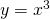and want to find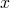or a well defined approximation ofwhen we know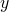. Mathematically speaking we want to assume thatis constant and we want to find anfor which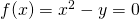or. If we guess such anand then draw the tangent at the curve of the function at the pointor, then the intersection point of the tangent can be used as the next approximation. This method converges in the case of these two functions (and some others) and is reasonably fast. Now the tangent has the linear equationwhere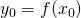and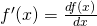is the derivative of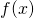. We want to solve this equation for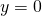and thus we get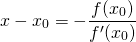and thus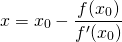As an iteration rule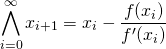In case of the sqare root we can just start with an estimation by shifting half the length to the right, but avoiding zero, which is important because of the division. Then we get for an appropriate n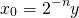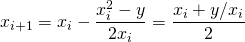The last form is quite intuitive, even without calculus. As I said this converges usefully fast and there is tons of math around to describe the behavior, speed, precision and convergence of the calculations performed in this algorithm. Written in Ruby just for integers, this is quite simple. Convergence is simply discovered by the fact that the result does not change any more, which may fail in some cases, where intermediate results oscillate between two values, but just for the purpose of benchmarking it seems to be sufficient:
 def sqrt_newton(x)   if (x == 0) then     return 0   end   y0 = x   u0 = x   while (u0 > 0) do     y0 >>= 1     u0 >>= 2   end   y0 = [1, y0].max   yi = y0   yi_minus_1 = -1   loop do     yi_plus_1 = (yi + x/yi) >> 1;     if (yi_minus_1 == yi_plus_1) then       return [yi, yi_minus_1].min     elsif (yi == yi_plus_1) then       return yi     end     yi_minus_1 = yi     yi = yi_plus_1   end end 

The newton algorithm tends to oscillate between two approximations, so this termination criteria takes into account,and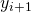and uses the lower of the two oscillating values. This results in calculating the largest integersuch that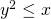and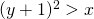.

For the third root we get for an appropriate n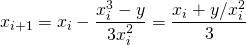Again this is a useful way and there is math around on when to stop for a desired precision.
Read Wikipedia for the convergence issues.

There is another approach, that people used to know when doing calculations on paper was more important than today. For the decimal system it works like this:
 1. 7 3 2 0 5 ---------------------- / 3.00 00 00 00 00 /\/ 1 = 20*0*1+1^2 - 2 00 1 89 = 20*1*7+7^2 ---- 11 00 10 29 = 20*17*3+3^2 ----- 71 00 69 24 = 20*173*2+2^2 ----- 1 76 00 0 = 20*1732*0+0^2 ------- 1 76 00 00 1 73 20 25 = 20*17320*5+5^2 ---------- 2 79 75 (source Wikipedia)
We group the digits to the left and to the right of the decimal point in groups of two. The highest possible square of an integral number that is below or equal to the leftmost group (03 in the example above) is used for the first digit of the result (1 in the example above). This square is subtracted and the next group is appended (200 in the example). Assuming thatis the result already calculated and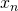is what we have achieved after the subtraction and the appending of the next group, we search for a digit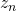such that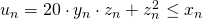.is chosen in such a way that it yields the maximum possiblewich is still. Subtractingfromand appending the next group allows for the next iteration.

Now this can be turned into an algorithm. The first approach is to just switch from decimal system to binary system. Then for each iteration step we have to deal just with the possible values of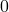and, which greatly simplifies the algorithm. Here is a simple ruby program that would do this:
 def split_to_words(x, word_len)   bit_pattern = (1 << word_len) - 1   words = []   while (x != 0 || words.length == 0) do     w = x & bit_pattern     x = x >> word_len     words.unshift(w)   end   words end

 

def sqrt_bin(x)   if (x == 0) then     return 0   end   xwords = split_to_words(x, 2)   xi = xwords - 1   yi = 1   1.upto(xwords.length-1) do |i|     xi = (xi << 2) + xwords[i]     d0 = (yi << 2) + 1     r  = xi - d0     b  = 0     if (r >= 0) then       b  = 1       xi = r     end     yi = (yi << 1) + b   end   return yi end 

It seems that the two solutions yield the same results, but the sqrt_newton outperforms sqrt_bin by a factor of two.

Now we should reconsider, if base 2 is really the best choice. Actually we can use any power of 2 as a base and efficiently work with that. Apart from the initial first step, which is done by using an extended version of sqrt_bin, the next steps are estimated by division and trying neighboring values to get the exact result. This makes use of the fact that the equation we need to solve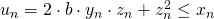with the maximumfullfilling this equation, where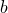is the base to which we are working, witch was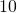or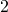above and could now be a power of. As soon as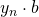has a certain size, the influence of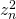becomes less relevant. We can consider the maximum posible value for z_n, which is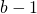and thus solve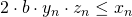and, each for the maximumfullfilling the equation. This can be calculated by simple division. If the range between the two solutions is small enough, then each value in the range can be tried to find the actual accurate solution for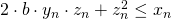and this is more efficient than working just bitwise. This method sqrt_word seems to outperform sqrt_newton for longer numbers, for example around 60 decimal digits with word_length=16. So the most promising approach seems to be to optimize the implementation and parameters of sqrt_word. The issue of termination, which has been properly addressed in the newton implementation, is already dealt with in this implementation. For more serious analysis it would be interesting to implement the algorithms in C or even in assembly language. So this is the final result for square roots, with some checks added:
 def check_is_nonneg_int(x, name)   raise TypeError, "#{name}=#{x.inspect} must be Integer" unless (x.kind_of? Integer) && x >= 0 end

 def check_word_len(word_len, name="word_len")   unless ((word_len.kind_of? Integer) && word_len > 0 && word_len <= 1024)     raise TypeError, "#{name} must be a positive number <= 1024"   end end def split_to_words(x, word_len)   check_is_nonneg_int(x, "x")   check_word_len(word_len)   bit_pattern = (1 << word_len) - 1   words = []   while (x != 0 || words.length == 0) do     w = x & bit_pattern     x = x >> word_len     words.unshift(w)   end   words end def sqrt_bin(x)   yy = sqrt_bin_with_remainder(x)   yy end def sqrt_bin_with_remainder(x)   check_is_nonneg_int(x, "x")   if (x == 0) then     return [0, 0]   end   xwords = split_to_words(x, 2)   xi = xwords - 1   yi = 1   1.upto(xwords.length-1) do |i|     xi = (xi << 2) + xwords[i]     d0 = (yi << 2) + 1     r  = xi - d0     b  = 0     if (r >= 0) then       b  = 1       xi = r     end     yi = (yi << 1) + b   end   return [yi, xi] end def sqrt_word(x, n = 16)   check_is_nonneg_int(x, "x")   check_is_nonneg_int(n, "n")   n2 = n << 1   n1 = n+1   check_word_len(n2, "2*n")   if (x == 0) then     return 0   end   xwords = split_to_words(x, n2)   if (xwords.length == 1) then     return sqrt_bin(xwords)   end   xi = (xwords << n2) + xwords   a  = sqrt_bin_with_remainder(xi)   yi = a   if (xwords.length <= 2) then     return yi   end   xi = a   2.upto(xwords.length-1) do |i|     xi = (xi << n2) + xwords[i]     d0 = (yi << n1)     q  = (xi / d0).to_i     j  = 10     was_negative = false     while (true) do       d = d0 + q       r = xi - (q * d)       break if (0 <= r && (r < d || was_negative))       if (r < 0) then         was_negative = true         q = q-1       else         q = q+1       end       j -= 1       if (j <= 0) then         break       end     end     xi = r     yi = (yi << n) + q   end   return yi end 

def sqrt_newton(x)   check_is_nonneg_int(x, "x")   if (x == 0) then     return 0   end   y0 = x   u0 = x   while (u0 > 0) do     y0 >>= 1     u0 >>= 2   end   y0 = [1, y0].max   yi = y0   yi_minus_1 = -1   loop do     yi_plus_1 = (yi + x/yi) >> 1;     if (yi_minus_1 == yi_plus_1) then       return [yi, yi_minus_1].min     elsif (yi == yi_plus_1) then       return yi     end     yi_minus_1 = yi     yi = yi_plus_1   end end 

This is the approach that has been built into the LongDecimal library, ignoring Newton. The examples have been added to github.

The algorithms can be extended to cubic roots or any higher roots. In this case, the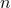th root ofis calculated by starting with the maximal integral number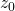with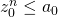and the subsequently finding numbers z_j fullfilling an equation of the form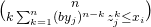. This is always easy to handle for base two, by just testing the two possible solutions. For higher bases and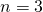it involves solving an quadratic equation, once the numbers are high enough to neglect the term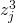. For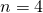it is just possible to take the square root of the square root. For higher values ofand bases other thanit becomes really difficult to tame this algorithm. So I intend to constrain myself to square roots and cube roots. I have not explored, if it is useful to calculate the cube root with a higher base thanand which approach provides the best performance for cube roots. Even the square root calculation can possibly be tuned a bit. Maybe this will be addressed in another article.

# Guava-Collections in Java-APIs

When we write APIs, that have parameters or as return values, it is a good idea to consider relying on immutable objects only. This applies also when collections are involved directly or indirectly as content of the classes that occur as return values or parameters. Changing what is given through the API in either direction can create weird side effects. It even causes different behavior, depending on weather the API works locally or via the network, because changes of the parameters are usually not brought back to the caller via some hidden back channel, unless we run locally. I use Java as an example, but it is quite an universal concept and applies to many languages. If we talk about Ruby, for example, the freeze method might be our friend, but it goes only one level deep and we actually want to deep-freeze.. Another story, maybe…

Now we can think of using Java’s own Collections.unmodifiableList and likewise. But that is not really ideal. First of all, these collections can still be modified by just working on the inner of the two collections:
 List list = new ArrayList<>(); list.add("a"); list.add("b"); list.add("c"); List ulist = Collections.unmodifiableList(list); list.add("d"); ulist.get(3) -> "d" 
Now list in the example above may already come from a source that we don’t really control or know, so it may be modified there, because it is just „in use“. Unless we actually copy the collection and put it into an unmodifiableXXX after that and only retain the unmodifiable variant of it, this is not really a good guarantee against accidental change and weird effects. Let’s not get into the issue, that this is just controlled at runtime and not at compile time. There are languages or even libraries for Java, that would allow to require an immutable List at compile time. While this is natural in languages like Scala, you have to leave the usual space of interfaces in Java, because it is also really a good idea to have the „standard“ interfaces in the APIs. But at least use immutable implementations.

When we copy and wrap, we still get the weird indirection of working through the methods of two classes and carrying around two instances. Normally that is not an issue, but it can be. I find it more elegant to use Guava in these cases and just copy into a natively immutable collection in one step.

For new projects or projects undergoing a major refactoring, I strongly recommend this approach. But it is of course only a good idea, if we still keep our APIs consistent. Doing one of 100 APIs right is not worth an additional inconsistency. It is a matter of taste, to use standard Java interfaces implemented by Guava or Guava interfaces and classes itself for the API. But it should be consistent.

# Scala Days 2019

Scala Days had a tenth anniversary in 2019. It is an annual conference about Scala, which I like to visit, when possible.

There is some confusion as to what is meant:

• Where this the 9th, 10th or 11th Scala Days?
• Where the first Scala Days 9, 10 or 11 years ago?

The truth is, that the first Scala Days were organized in 2010 and took place in Lausanne. So it was 9 years ago, but the tenth event. For this reason it was done in Lausanne again, but in a much larger venue.

Just for the completeness:

This is the opening keynote by Martin Odersky. A video will be on youtube soon.

I visited the following talks:

And on the last day I visited the following talks and the diversity panel and the closing panel:

Here is the whole schedule.

Btw., links might expire when the next Scala Days will start using the site in early 2020. Where it will be in 2020 is still unknown, but since 2014 it has been a habit to do the even years in Berlin. I will try to come, if there are Scala Days 2020 in Europe.

# hashCode, equals and toString

In many programming languages we are urged to define methods hashCode, equals and toString. They are named like this in Java and in many JVM languages or they use similar names. Some languages like Perl and Scala provide decent mechanisms for the language to figure these out itself, which we do most of the time in Java as well by letting the IDE create it for us or by using a library. This solution is not really as good as having it done without polluting our source code and without using mechanisms like reflection, but it is usually the best choice we have in Java. It does have advantages, because it gives us some control over how to define it, if we are willing to exercise this control.

So why should we bother? equals is an „obvious“ concept that we need all the time by itself. And hashCode we need, when we put something into HashMaps or HashSets or something like that. It is very important to follow the basic contract, that hashCode and equals must be compatible, that is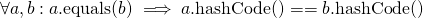And equals of course needs to be an equivalence relation.
There has been an article in this blog about „Can hashCodes impose a security risk?„, which covers aspects that are not covered here again.

An important observation is that these do not fit together with mutability very well. When we mutate objects, their hashCode and equals methods yield different results than before, but the HashSet and HashMap assume that they remain constant. This is not too bad, because usually we actually use very immutable objects like Strings and wrapped primitive numbers as keys for Maps. But as soon as we actually write hashCode and equals, this implies that we are considering the objects of this type to be members of HashMaps or HashSets as keys and the mutability question arises. One very ugly case is the object that we put into the database using Hibernate or something similar. Usually there is an ID field, which is generated, while we insert into the database using a sequence, for example. It is good to use a sequence from the database, because it provides the most robust and reliable mechanism for creating unique ids. This id becomes then the most plausible basis for hashCode, but it is null in the beginning. I have not yet found any really satisfying solution, other than avoiding Hibernate and JPAx. Seriously, I do think, that plain JDBC or any framework like MyBatis or Slick with less „magic“ is a better approach. But that is just a special case of a more general issue. So for objects that have not yet made the roundtrip to the database, hashCode and equals should be considered dangerous.

Now we have the issue that equality can be optimized for hashing, which would be accomplished by basing it on a minimal unique subset of attributes. Or it could be used to express an equality of all attributes, excluding maybe some kind of volatile caching attributes, if such things apply. When working with large hash tables, it does make a difference, because the comparison needs to look into a lot more attributes, which do not change the actual result at least for each comparison that succeeds. It also makes a difference, in which order the attributes are compared for equality. It is usually good to look into attributes that have a larger chance of yielding inequality, so that in case of inequality only one or only few comparisons are needed.

For the hashCode it is not very wrong to base it on the same attributes that are used for the equals-comparison, with this usual pattern of calculating hash codes of the parts and multiplying them with different powers of the some two-digit prime number before adding them. It is often a wise choice to chose a subset of these attributes that makes a difference most of the time and provides high selectivity. The collisions are rare and the calculation of the hash code is efficient.

Now the third method in the „club“ is usually toString(). I have a habit of defining toString, because it is useful for logging and sometimes even for debugging. I recommend making it short and expressive. So I prefer the format
className(attr1=val1 attr2=val2 att3=val3)
with className the name of the actual class of this object without package, as received by
getClass().getSimpleName()
and only including attributes that are of real interest. Commas are not necessary and should be avoided, they are just useless noise. It does not matter if the parantheses are „()“ or „[]“ or „{}“ or „«»“, but why not make it consistent within the project. If attribute values are strings and contain spaces, it might be a good idea to quote them. If they contain non-printable characters or quotation marks, maybe escaping is a good idea. For a real complete representation with all attributes a method toLongString() can be defined. Usually log files are already too much cluttered with noise and it is good to keep them consise and avoid noise.

# ScalaUA 2019

In March 2019 I have visited ScalaUA in Kiev.

It was interesting. I attended the following talks:

# Unicode and C

It is a common practice in C to use arrays of char as strings. The 0 is used as end marker.

The whole thing was created like that in the 1970s and at that time it was kind of cool to get away with one less language feature and to express it in terms of others instead. And people did not think enough about the necessity to express more than ISO 646 IRV (commonly called ASCII) as string content.

This extended out of the box to 8 bit character sets like ISO 8859-1 or KOI-8, that are identical to ISO 646 in the lower 128 characters and contain an extension in the upper 128 characters. But fortunately we have moved ahead and now Unicode with its encodings UTF-8, UTF-16 and UTF-32.

How can we deal with this in C?

UTF-8 just works out of the box, because the byte 0 is only used to encode the code point U+0000. So the null termination can be kept as it is and a lot of functionality remains valid. Some issues arise, because in UTF-8 things like finding the logical length of a string, not its memory consumption or finding the nth code point, not the nth byte, require UTF-8-logic to be applied and to parse the whole string at least from the beginning to the desired position or the usage of an indexing facility. So a lot of non-trivial string functionality of the standard library will just not be as easy as people thought it would be in the 1970s and subsequently not work as needed. Libraries for better UTF-8-support in C can be found, leaving the „native“ C strings with UTF-8 content only for usage in interfaces that require them. I have not yet explored such libraries, but it would be interesting to find out how powerful and useful they are.

At the time when Unicode came out, it seemed to be sufficient to have 16 bits per character instead of 8. Java was built on this assumption. C added a wchar_t to allow for this and just required it to be „long enough“. So Linux uses 32 bits and MS-Windows 16 bits. This is not too bad, because programming in C for MS-Windows and for Linux is anyway quite different, unless we abstract the differences into a library, which would then also include a common string definition and string handling functionality. While the Linux wchar_t is sufficient, it really wastes a lot of memory, which is often undesirable, if we go the extra effort to program in C in order to gain performance. The Windows-wchar_t is „kind of sufficient“, as are the Java-Strings, because we can really do a lot with assuming that Unicode is only 16 bit or with UTF-16 and ignoring the complexities of that, that are in principal the same as for UTF-8, but can be ignored with less disadvantages most of the time. The good news is, that wchar_t is well supported by standard library functions.

Another way is to use char16_t and char32_t, that have a clear definition of their length, but much less library support.

Probably these facilities are sufficient for software whose string handling is relatively trivial. For more ambitious string handling in terms of functionality and performance, it will be necessary to find third party libraries or to write them.

# Flashsort in Scala

There is now also an implementation of Flashsort in Scala.

In order to solve the requirement of sorting part of an array that is needed as part of flashsort, an heapsort implementation in Scala that can be constrained to a part of an array has been included as well. Heapsort was chosen, because it can sort in place and it has a guaranteed performance of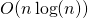. Mergesort or quicksort would have been reasonable choices as well. Some implentations even use insertion sort for this step, because the sections are small.

# Flashsort in Ruby

Deutsch

There is a simple implementation of Flashsort in Ruby, after having already provided an implementation in C. The C-implementation is typically faster than the libc-function qsort, but this depends always on the data and on how well the metric-function has been written, that is needed on top of the comparison function for Flashsort. You can think of this metric function as some kind of monotonic hash function. So we have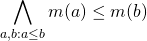This additionally needed function of method is not really there, apart from numerical values, so we really have to invest some time into writing it. This makes the use of Flashsort a bit harder. A good metric function is crucial for good performance, but for typical text files quite trivial implentations already outperform classical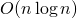algorithms like Heapsort and Quicksort and Mergesort for larger amounts of data.

This blog article shows other sorting algorithms for Ruby.